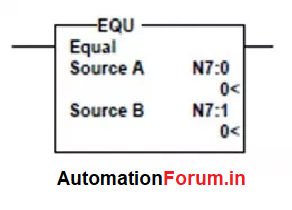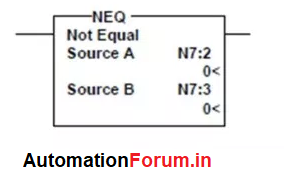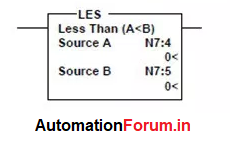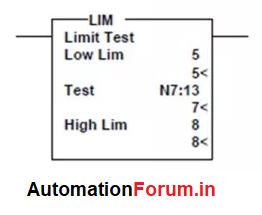# PLC tutorial - Compare instructions

## What are compare instructions?

These are input instructions that test the relationship between two values from two different sources. A compare instruction specifically tests the following sources:

• Source A: a word address.
• Source B: another word address or constant.

When the logic comparison is true, the rung will be true and the output will be set to 1.

There are different compare instructions listed below:

## EQU (Equal) instructionAn input instruction that tests whether the two user-specified values are the same. If the value is equal, meaning the instructions are logic true. If two values are not the same, it means that the logic instructions are false.

## NEQ (Not Equal) instruction:An input instruction that tests whether two user-specified values are not the same. If Source A and Source B are not the same, the instructions are logic true. If two values are the same, the logic instruction is false.

## LES (Less Than) instruction:A condition of input instruction that tests whether a value is less than the second value. If the Source A value is less than the value of Source B, the logic instruction is true. If the value in Source A is greater than or equal to the value in Source B, the logic instruction is false.

## LEQ (Less Than or Equal) instruction:

A condition of input instruction that tests whether a value is less than or equal to the second value. If the value in Source A is less than or equal to the value in Source B, the logic instruction is true. If the value in Source A is greater than the value in Source B, the logic instruction is false.

## GRT (Greater Than) instruction:

An input instruction that tests whether a value is greater than the second value. If the Source A value is greater than Source B, the logic instruction is true. If the value in Source A is smaller with the value in Source B, the logic instruction is false.

## GEQ (Greater Than or Equal) Instructions:

An input instruction that tests whether one value is greater than or equal to a second value. If the value stored in Source A is greater or equal to the value stored in Source B, then rung becomes true. If the value in Source A is less than the value from Source B, the logic instruction is false.

## LIM (Limit Test) Instructions:An input instruction that tests whether a value between or outside the limit is defined.

1 Like